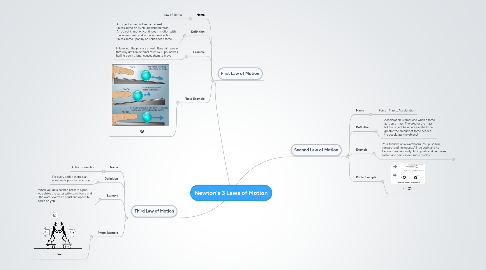# Newton's 3 Laws of Motion

Get Started. It's FreeNewton's 3 Laws of Motion## 1. First Law of Motion

### 1.1. Name

1.1.1. Law of Inertia

### 1.2. Definition

1.2.1. An object at rest will remain at rest unless acted on by an unbalanced force. An object in motion continues in motion with the same speed and in the same direction unless acted upon by an unbalanced force

### 1.3. Example

1.3.1. In bowling, the pins are at rest. They will remain that way until an external force (a 15 pound ball hurling down a lane) causes them to move

1.4.1. -

## 2. Second Law of Motion

### 2.1. Name

2.1.1. Force= Mass x Acceleration

### 2.2. Definition

2.2.1. Acceleration is produced when a force acts on a mass. The greater the mass (of the object being accelerated) the greater the amount of force needed (to accelerate the object).

### 2.3. Example

2.3.1. Your friend is on a skateboard. You push him forward, and he moves. A little push, and he begins to move slowly. A big push, and he moves faster, and gains speed more quickly.

2.3.1.1. New node

2.4.1. -

## 3. Third Law of Motion

### 3.1. Name

3.1.1. Action + Reaction

### 3.2. Definition

3.2.1. For every action there is an equal and opposite re-action

### 3.3. Example

3.3.1. When you do a cannon ball into a pool, you strike the water with some force and the water exerts an equal and opposite force on you

3.4.1. -